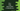# C++ program to find the sum of first n natural numbers## C++ program to find the sum of first n natural numbers :

In this C++ program, we will learn how to find the sum of first n natural numbers. The program will ask the user to enter the value of n and it will find the result. We will learn three different ways to solve this problem. This is a beginner level C++ program and you will learn how to take user input, how to use loops and how to do mathematical calculations with this program.

### Method 1: By using a for loop:

We can use one for loop to iterate from 1 to n and find out the sum of all the numbers. Below is the complete program:

``````#include <iostream>
using namespace std;

int main() {
int n;
int sum = 0;

cout << "Enter the value of n :"<<endl;
cin >> n;

for(int i = 1; i<= n; i++){
sum += i;
}

cout << "Total sum :"<<sum<<endl;
return 0;
}``````
• n is the variable we are storing the user provided value.
• sum variable is used to store the sum. Its initial value is 0.
• The for loop runs from i = 1 to i = n.
• Inside the loop, we are adding the current value of i to sum. i.e. we at the end of the loop, sum holds the sum of 1 to n.
• Finally, we are printing the total sum i.e. sum

### Sample Output:

``````Enter the value of n :
10
Total sum :55``````

### Method 2: By using a while loop:

Similar to a for loop, we can also use one while loop to find out the sum. while loop checks one condition and runs the loop continuously till the condition is true. If we write the above program using while loop, it looks as like below:

``````#include <iostream>
using namespace std;

int main() {
int n;
int sum = 0;
int i = 1;

cout << "Enter the value of n :"<<endl;
cin >> n;

while(i <= n){
sum += i;
i++;
}

cout << "Total sum :"<<sum<<endl;
return 0;
}``````

Here, we are defining one variable i with value 1. The while loop runs and increment the value of sum on each iteration. It prints similar output as the above example.

### Method 3: By using mathematical formula:

The sum of n natural numbers is n*(n + 1)/2. Instead of using a loop, we can directly use this formula. This will be much faster because we don’t have to use the loop. For example, if we have to find the sum of first 1000 numbers, the loop will have to run 1000 times to find out the sum. But if we use the mathematical formula, it will find it immediately.

``````#include <iostream>
using namespace std;

int main() {
int n;
int sum;

cout << "Enter the value of n :"<<endl;
cin >> n;

sum = n*(n + 1)/2;

cout << "Total sum :"<<sum<<endl;
return 0;
}``````

It will give similar outputs as the above programs.